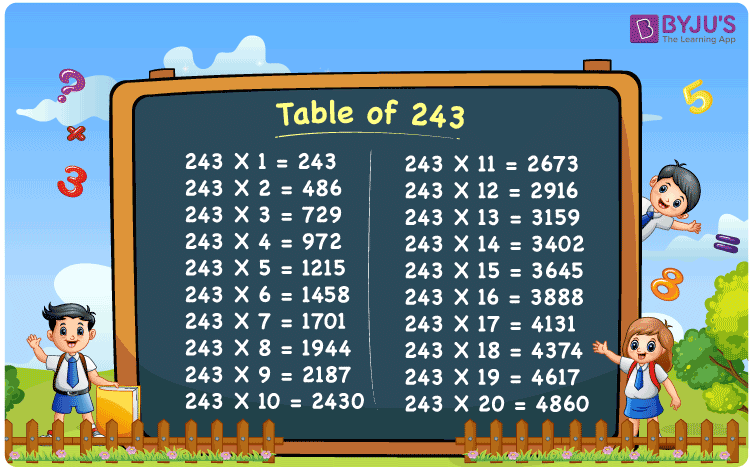Checkout JEE MAINS 2022 Question Paper Analysis : Checkout JEE MAINS 2022 Question Paper Analysis :

# Table of 243

The table of 243 is the multiplication table of 243 that can be written using the multiplication of 243 by numbers 1, 2, 3, and so on. As we know, maths tables are a useful resource for the students since these can be applied to simplify the numerical calculations. In this article, you will learn the table of 243, how to create the 243 times table using addition and multiplication, along with solved examples.

## Table of 243 Chart## What is the 243 Times Table?

The 243 times table is given below that is generated through the repeated addition of 243 and the multiplication of 243 by numbers 1, 2, 3, 4, 5, etc.

 Using multiplication Using repeated addition 243 × 1 = 243 243 243 × 2 = 486 243 + 243 = 486 243 × 3 = 729 243 + 243 + 243 = 729 243 × 4 = 972 243 + 243 + 243 + 243 = 972 243 × 5 = 1215 243 + 243 + 243 + 243 + 243 = 1215 243 × 6 = 1458 243 + 243 + 243 + 243 + 243 + 243 = 1458 243 × 7 = 1701 243 + 243 + 243 + 243 + 243 + 243 + 243 = 1701 243 × 8 = 1944 243 + 243 + 243 + 243 + 243 + 243 + 243 + 243 = 1944 243 × 9 = 2187 243 + 243 + 243 + 243 + 243 + 243 + 243 + 243 + 243 = 2187 243 × 10 = 2430 243 + 243 + 243 + 243 + 243 + 243 + 243 + 243 + 243 + 243 = 2430

## Multiplication Table of 243

Go through the multiplication table of 243 given here to learn the table of 243 up to 20 results.

 243 × 1 = 243 243 × 2 = 486 243 × 3 = 729 243 × 4 = 972 243 × 5 = 1215 243 × 6 = 1458 243 × 7 = 1701 243 × 8 = 1944 243 × 9 = 2187 243 × 10 = 2430 243 × 11 = 2673 243 × 12 = 2916 243 × 13 = 3159 243 × 14 = 3402 243 × 15 = 3645 243 × 16 = 3888 243 × 17 = 4131 243 × 18 = 4374 243 × 19 = 4617 243 × 20 = 4860

## Examples on the Table of 243

Example 1:

How many wheels do 243 bicycles have?

Solution:

We know that a bicycle contains 2 wheels.

Number of bicycles = 243

Total number of wheels = ?

Using the table of 243, 243 × 2 = 486

Therefore, the total number of wheels = 486.

Example 2:

There are 243 flower vases, and each flower vase contains 8 flowers. Find the total number of flowers.

Solution:

Number of vases = 243

Number of flowers in each flower vase = 8

Total number of flowers = 243 × 8 = 1944.

## Frequently Asked Questions on Table of 243

### What is the table of 243?

The table of 243 is the set of multiplication results when 243 is multiplied by natural numbers. Thus, the multiplication table of 243 can be written as:
243 × 1 = 243
243 × 2 = 486
243 × 3 = 729
243 × 4 = 972
243 × 5 = 1215
243 × 6 = 1458, etc.

### In which tables 243 will come?

In the following tables, 243 will come as a result.
In the 3 times table, 3 × 81 = 243
In the 9 times table, 9 × 27 = 243

### Using the table of 243, find the value of 243 times 15.

Using the table of 243, 243 times 15 = 243 × 15 = 3645.

### How do you calculate the value of 243 times 7?

The value of 243 times 7 can be calculated using the 243 times table as shown below: 243 times 7 = 243 × 7 = 1701
Or
243 + 243 + 243 + 243 + 243 + 243 + 243 = 1701